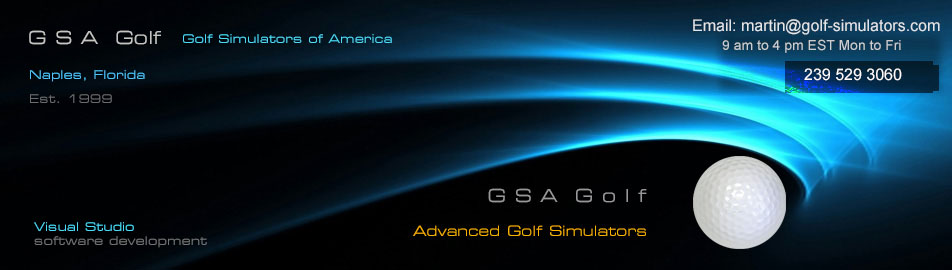LX Pro Launch monitor

Operating & Installation instructions

Part 2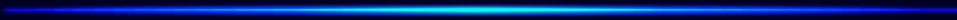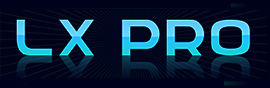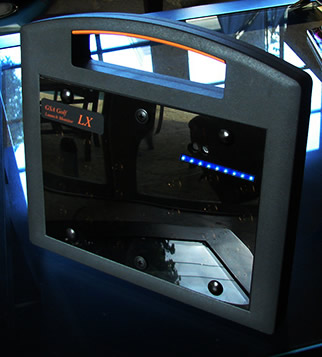Ball markings

To-date, all camera based launch monitors require some sort of markings on the ball to detect spin and spin axis in real-time.

Real-time meaning that there is no or little detectable lag time between the time of the club to ball impact and the time the ball starts to launch in the game software with the measured ball spin.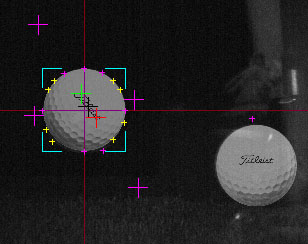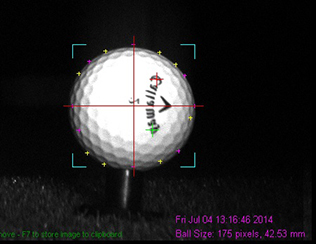Most golf balls usually have logos on them and as long as the logo clearly defined on the ball image, the LX can use it to measure the spin and spin axis.

Note that not all logos work as well as others. The "Titleist" logo for example is often too fine whereas the "Callaway" is far better.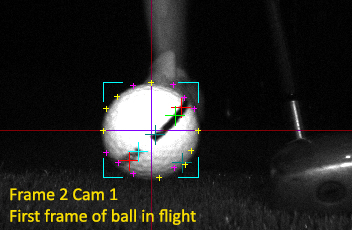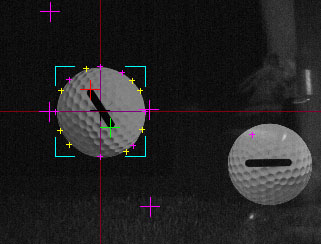Range balls often have a very well defined line on them and usually work better than most logos but there is a disadvantage.

Namely: if the line is too long then it is quite possible that not both ends of the line are visible to the camera.

The system requires that both ends of the line or logo are visible in all frames in order to calculate spin axis.

The ball line in the above left image for example is too long whereas the ball line in the above right image is just right.For best results, we're now recommending balls similar to the "Pinnacle" or "Srixon" distance balls. These balls have a shorter logo and the number and other markings are red so don't are not seen by the gray level cameras.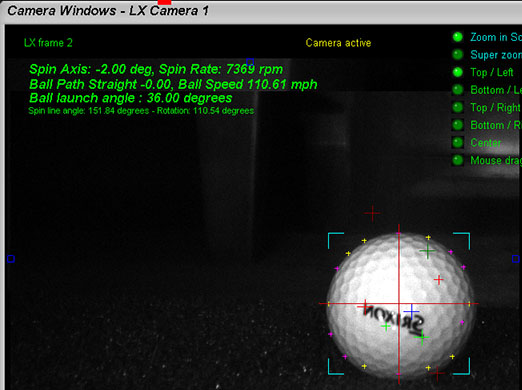Here, a shot of the Srixon "Distance" ball with the LX showing the strong lettering being easily detected and the red ball number not visible.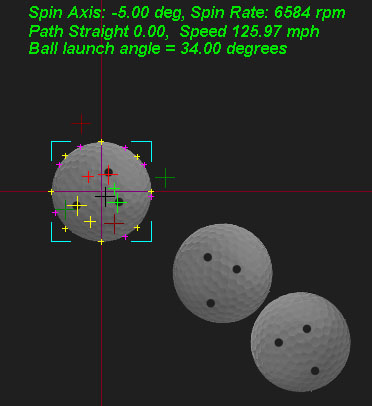Ideally the ball should have 3 or more dots on it. The reasons for this are twofold:

1. Logos and lines have only 2 ends and it is sometimes difficult to determine which end is which.

Not knowing which end is which can lead to incorrect spin and spin axis readings. i.e. has the ball rotated more than or less than 180 degrees?

With 3 or more dots placed on the ball unsymmetrically , the dots form a pattern that can always be located no matter how many degrees the ball has rotated.

2. Logos and lines are usually only on either 1 or 2 sides of the ball

which then requires the player to have to bend down and place the ball in front of the unit so that the line of logo is facing the cameras in the unit for every shot where spin data is required.

Dots can be placed all around the ball so that the player can move the ball to the hitting position with a club or his foot. i.e. You'd only need to bend down when teeing up.

Spin dot balls

The LX Pro is now supplied with 12 spin dot golf balls.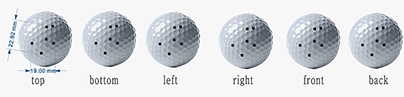Using these high quality tournament ball saves you from having to bend down and align the ball markings to the camera.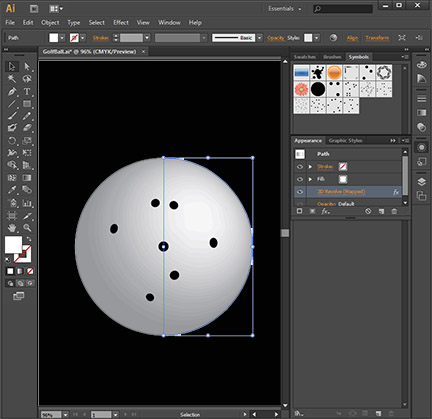The dots are bake printed in 6 positions on the golf balls (top, bottom, left, right, back and front) and will eliminate the requirement to manually align ball logos to the LX cameras.

12 balls are free of charge to all LX customers. Additional balls are \$4 each.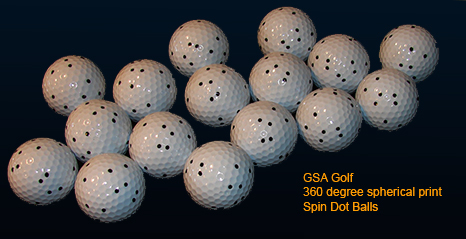When using the new spin dot balls, ensure that "Spin dots on ball" has been selected on the CP's main window and not "Ball line or Logo on ball".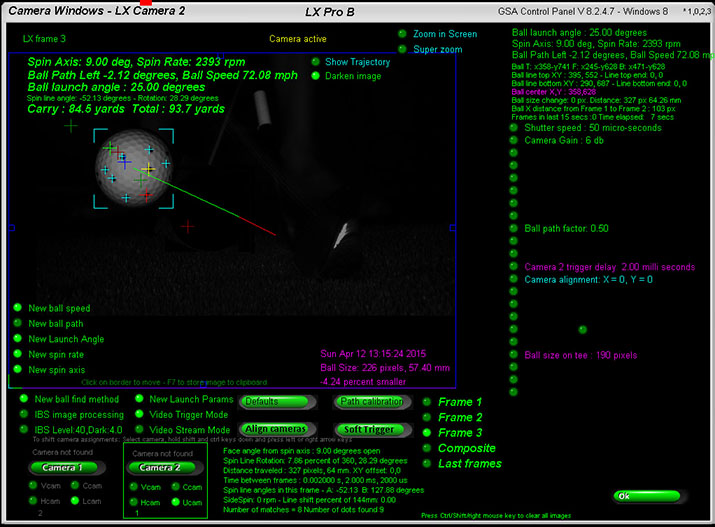LX Pro Testing

You can test the LX Pro's functionality with just the Control Panel running. i.e. without any golf course game software running.

The object of the tests is to see that the ball and spin line or ball logo is being correctly detected in each of the 3 frames after a shot with your camera settings.

Take shots with just the CP's main window open.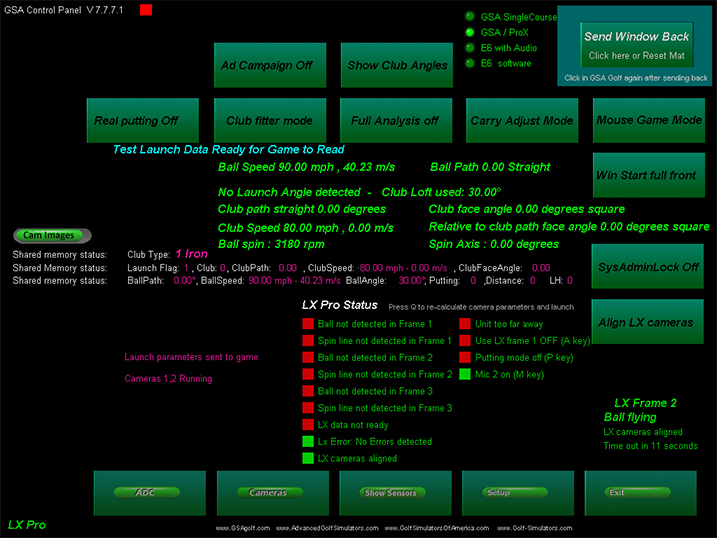After the shot, you should see that all the status boxes turn green and the "Ball flying" message is displayed.

(The red box on in the above image was just a test and would normally also be green)

Note. The "Ball flying" status will time out in about 10 seconds if game software is not running and the system will start looking for a ball on the mat again.

In this case the images of the last shot will be overwritten so you should open the camera window before this time out.

Verifying LX data

1. Verifying launch angle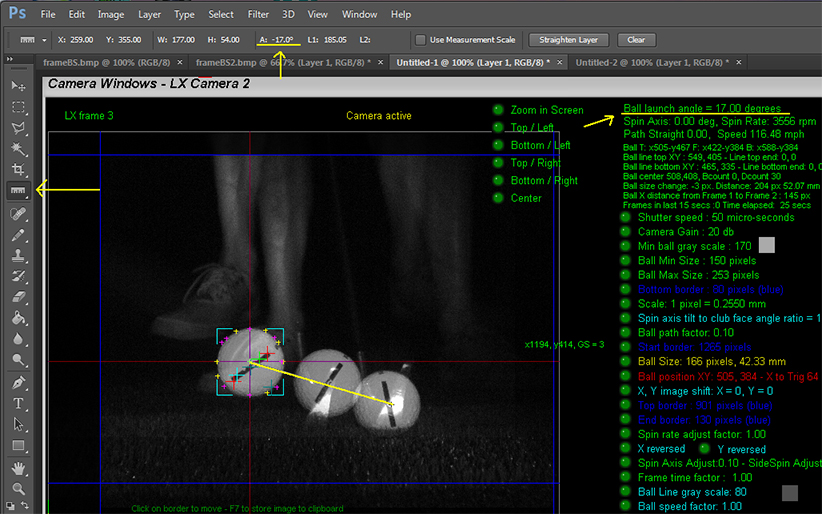This is the easiest parameter to verify. Obviously you have photographic evidence anyway but just to be sure use the following method:

1. After a shot, go to the Cameras window in the Control Panel, select camera 2 and select Composite. Your image should look similar to the one above.

2. Press the Prt Sc key on your PC's keyboard to copy a screen shot of the image to the Windows clipboard and paste in into a graphics tool like Photoshop

3. Launch angle in the LX Pro is measure from the center of the ball in frame 1 and the center of the ball in frame 3.

4. Using the Photoshop's measuring tool (right click on the EyeDrop tool to select this) set the points to the center of the ball's in frame 1 and 3. You should then see that the measured angle in Photoshop (shown at the top tool bar) corresponds to the measure launch angle in the LX's control panel.

2. Verifying ball speed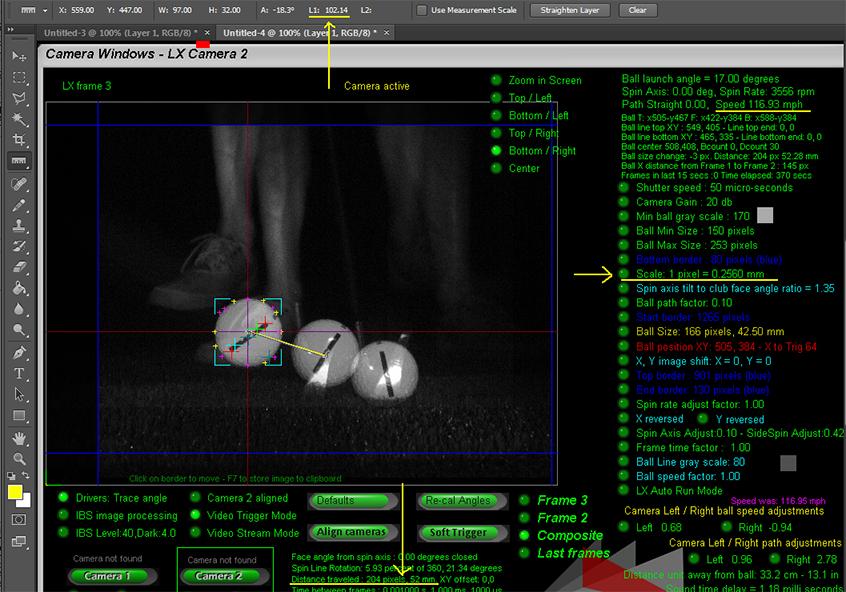Ball speed in the LX Pro is measured by the distance the ball traveled between frame 2 and frame 3 and the time taken to travel this distance.

The equation speed = distance / time is used.

Time is the constant electronic time delay between frame 2 and frame 3 of 1 millisecond. (or 0.001 seconds).

The unit of measure for a distance in the frame image is in pixels and this has to be converted to real life distances.

As we know the diameter a golf ball (42.67 mm) and can measure the diameter of the ball image in pixels, a scaling factor can be calculated.

The ball size (or diameter) in the above image is 166 pixels so if we divide the ball size in mm by this we get a scaling factor 42.67 / 166 = 0.257

The LX Pro in the above image has measured that the ball has traveled 204 pixels. Using the scaling factor we get a real distance of 52.48 (or 52 mm rounded).

You can use the same measuring method in Photoshop to verify that the distance measured in the LX is the same in the image.

Note that the frame images in the Control Panel without zoom on have been reduced by 50% in order to fit the image in the screen so you have to double the Photoshop distance to get the real distance in pixels.

In the above image, the Photoshop measured distance is 102 pixels. If you double this then you get the 204 pixels measured in the Control Panel.

So now, using a calculator and using the equation speed = distance / time we get (52mm/1000) / 0.001 second = 52 meters per second.

Multiply this by 2.237 to convert from meters per second to mph and we get 117 mph

(116.96 mph is rounded)

3. Verifying spin rate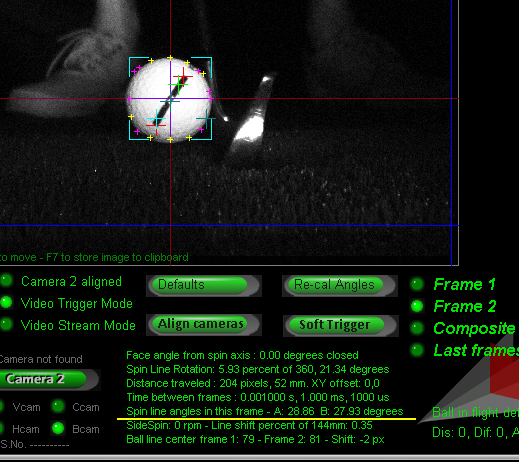--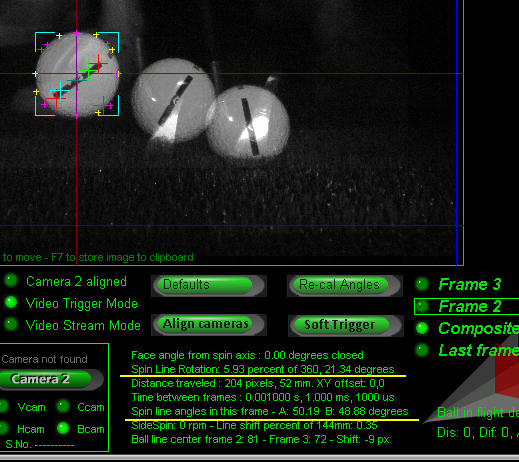Spin rate is measured by comparing the ball line angles in frame 2 and frame 3.

If we subtract the angle in frame 2 from the angle in frame 3 then we'll have the number of degrees of rotation within the 1 millisecond time frame.

For increased accuracy the image processing makes two spin line angle measurements: A and B.

Angle A cross hairs are in red and angle B cross hairs are in cyan.

In the above example, frame 2's spin line angles were 28.86 and 27.93 degrees and frame 3's spin line angles were 50.19 and 48.88 degrees

Subtracting A angles we get 50.19 - 28.86 = 21.33 degrees

(Rounding in the above example accounts for the 0.01 difference. i.e. 21.34 instead of 21.33)

If we know the ball rotated 21.34 degrees in the 1 millisecond frame time we can calculate the time it would take to rotate 1 degree in that time by dividing the 1 millisecond by the number of degrees of rotation.

So time required for 1 degree of rotation is 0.001 / 21.33 = 0.00004689 seconds

As there are 360 degrees in a complete rotation we multiply this time by 360 to give 0.01687 seconds.

So we have one complete 360 rotation every 0.01687 seconds which equates to 59.27 rotations (1/ 0.01687) every second and 3556 (59.27 * 60) rotations in one minute.

Spin rate in rpm is thus 3556 for this shot

Spin Axis detection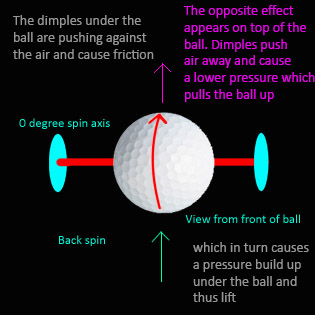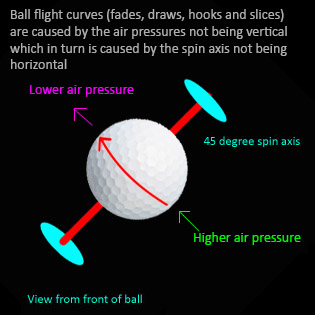In oder for the LX to detect spin axis in addition to spin rate, the complete logo or markings on the ball must be visible in all frames.

Thus, using a ball with a line or logo markings that go all around won't work as we need to see both ends of the markings in order to determine the center of rotation.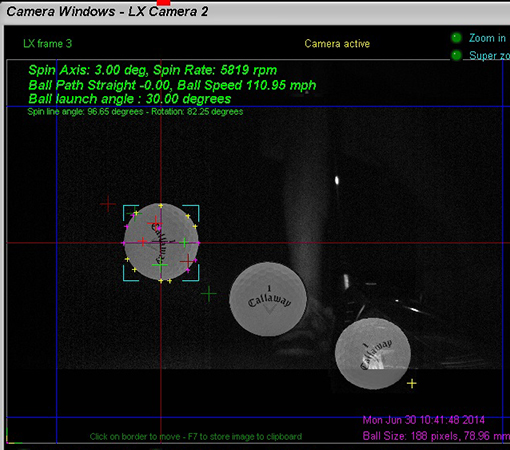Spin Axis detection theory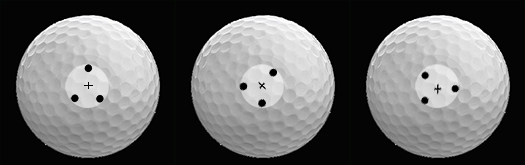Using 3 dots that form an isosceles triangle - ie a triangle with only two sides equal so that we can see the rotation angle - both spin rate and spin axis can be calculated.

The above image shows a ball spinning on a perfect horizontal axis with the dots at the center of rotation. With this - albeit rather unlikely scenario - the dot rotation is exactly in the center of the ball and thus it can be easily determined that the spin axis is horizontal.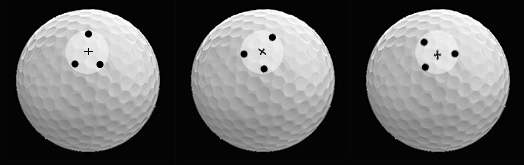If the dots are always in the center of rotation - again extremely unlikely - then calculating spin axis would be a simple matter. i.e. we just have to measure the center of rotation offset to the center of the ball and apply this to some simple trigonometric math to determine the spin axis.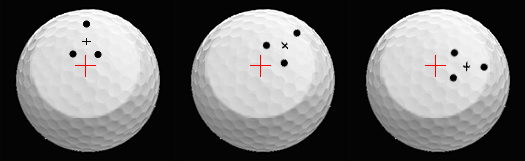The more likely scenario is that the spin dots are way off the center of rotation but - as shown above - it is still possible to determine the center of rotation by following the rotational path of the dots.

Again, once the center of rotation has been determined and it's distance away from the center of the ball is calculated, we can determine the spin axis.

Above image shows latest results of the new dot triangle method of detecting the spin and spin axis of the ball. Center of rotation is shown with the black cross hairs.

Red and green cross hairs have been programmed to not only find the dots but to also find the center of the dots. The pink cross hair shows the perpendicular to the triangle.

The black cross hair is the position of the intersection of the the triangle's perpendiculars in frames 1 and 2. This is then the center of rotation.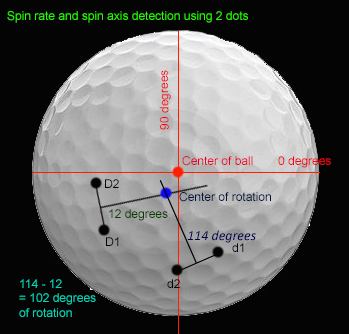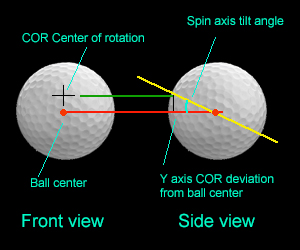Once the center of rotation has been detected, simple trigonometry is used to calculate the spin axis.-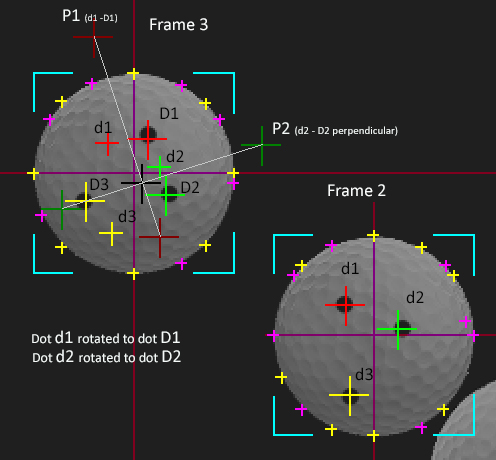LX cross hairs explained: The above images show dots 1,2 and 3 in frames 2 and 3. The red cross hairs are always dot 1, the green dot 2 and yellow dot 3.

All spin calculations are done in frame 3. The smaller red, green and yellow cross hairs in frame 3 show where the dots were in frame 2. The larger and fainter red and green cross hairs show the perpendiculars of the dot sets.

The point where these perpendiculars intersect is shown as a black cross hair. This is the center of rotation of the dots on the ball. From this intersect point we can calculate the spin axis.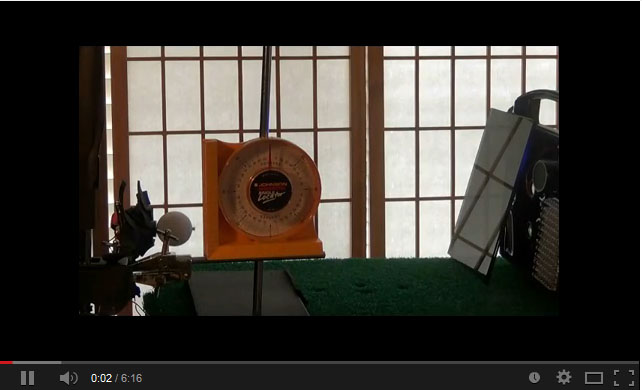LXPro Ball spin and spin axis lab / bench test video number 2.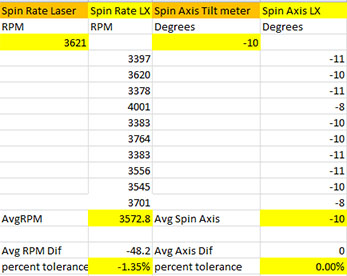-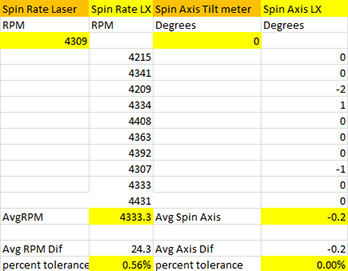Latest test figures now showing very expectable accuracy in both spin rate (rpm) and spin axis tilt (degrees).

4. Verifying ball path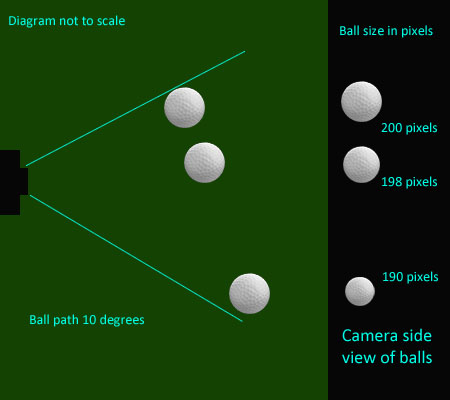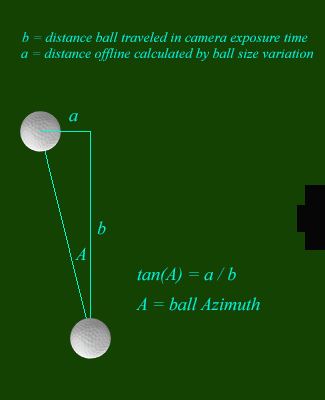Calculating ball path with a floor side mounted camera launch monitor is done by measuring the ball image size variation between the frames. ie. if the ball is traveling away from the camera then it's image size will appear smaller and if traveling towards the camera it's image size will appear larger. Thus ball image size variation is proportional to it's trajectory path direction.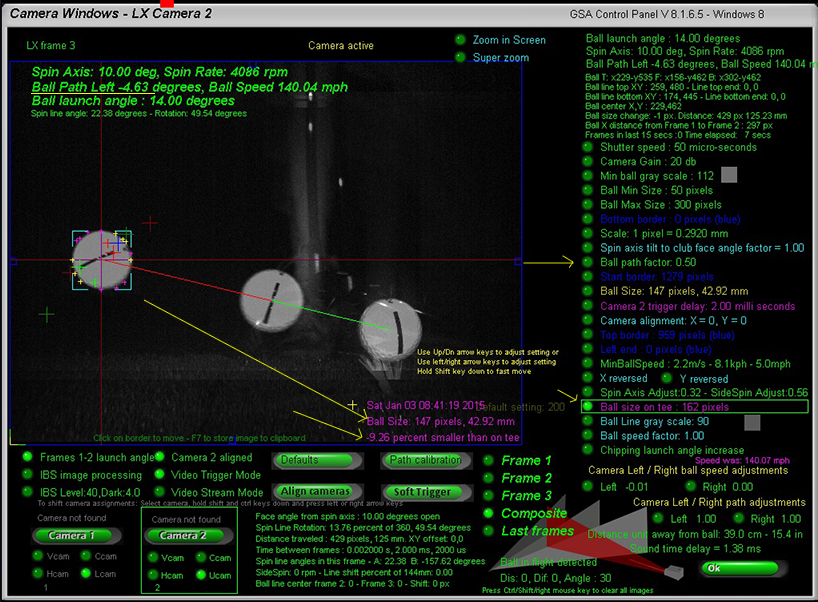--

The LX features two methods to calculate the ball direction from ball size variation: A percentage image size variation and a calibrated method.

The percentage size variation method uses the ball size as measured on the tee in frame 1 (this is automatically measured when aligning the cameras and stored) and the ball size in frame 3.

Tests have shown that the percentage of size variation is approximately 50% of it's path in degrees, so we simply multiply this percentage by a factor to get to degrees of ball path.

The user adjustable factor (Ball path factor) can be set by the user to influence the calculation.

Increasing this factor will result in greater ball path angles(less forgiving) and decreasing it will result in smaller ball path angles (more forgiving).

In the above example image the ball size on the tee was 162 pixels and in frame 3 (after the shot) it's size decreased to 147 pixels. A 9.26 percent variation in ball size.

As the ball image is smaller, we know that the ball had traveled away the camera and so was traveling left (assuming a right handed player).

With the ball path factor set to 0.50 in the above example, the ball path in degrees is thus 4.63 degrees left - (9.26 * 0.50 = 4.63).

Note that you can manually adjust the "Ball size on tee" i.e. the reference ball size.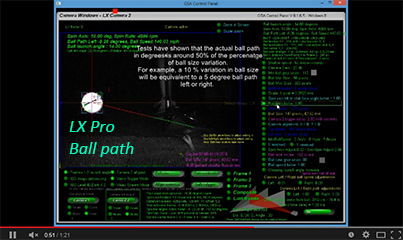Click above to see the Ball Path video

(The calibrated method will be explained at a later date)

LX Pro Setup quick check list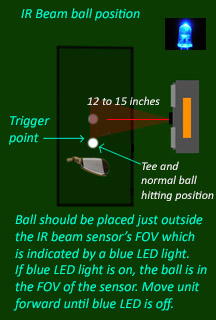1. Position Ensure that the unit is placed at the correct distance from the ball (12 to 15 inches)

and in the correct position (in line with the middle of the first bank of flash LEDs )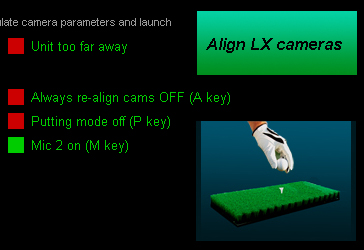2. Cameras alignment. The LX Pro features two cameras that are factory aligned but you can also manually re-align the cameras if desired.

To align the cameras, place a ball on a tee in it's usual tee position in front of the LX Pro and click the Align LX Cameras button.

You should see an animation indicating the alignment process which takes about 3 seconds..

When complete, the system will switch to frame 2 and be in the "Waiting for shot" mode.

Camera alignments are stored so if after use the unit is not moved the alignment process does not have to be repeated.

In addition to the camera alignment, the ball image size is stored which is used to calculate the ball path by comparing with the size of ball image in flight.3. Trigger mat IR Beam / Mic sensitivity and switch settings

For standard Trigger mat IR Beam trigger, switch the "Line scan camera - Mic/Ext" slide switch on the back of the unit to "Line scan camera" and the the "Mic Ext" slide switch to Ext.

Normally, the LX Pro will only be using the Trigger mat to trigger the cameras, however, you can also use a combination of both Trigger mat and Mic triggers if ambient lighting conditions are such (ie too light) that the IR beam sensor sensitivity can't be set high enough to capture fast shots. In this case - and because a fast full shot will produce a very distinct and load club to ball impact sound - the mic can be used as a back-up trigger. i.e. if the IR Beam trigger didn't detect the ball, then the mic will.

The microphone trigger can be switched on or off via the Control Panel's main screen.

Set the MIc 2 sensitivity (top dial on the back of the unit) so that it detects club/ball impact sound levels and not so sensitive that is picks up voices.

The sound level of clapping hands near the unit should be sufficient. If it triggers then the blue LED will light up briefly.

If the blue LED is continuously on then either the mic or the line scan camera's sensitivity is set too high.

If you only want to use the mic trigger, then you can switch the mic off via a button in the CP's main window.

4. Camera settings

"Right" mouse click on the "Defaults" button in the camera window of the CP. This will set all camera settings to their default factory settings.